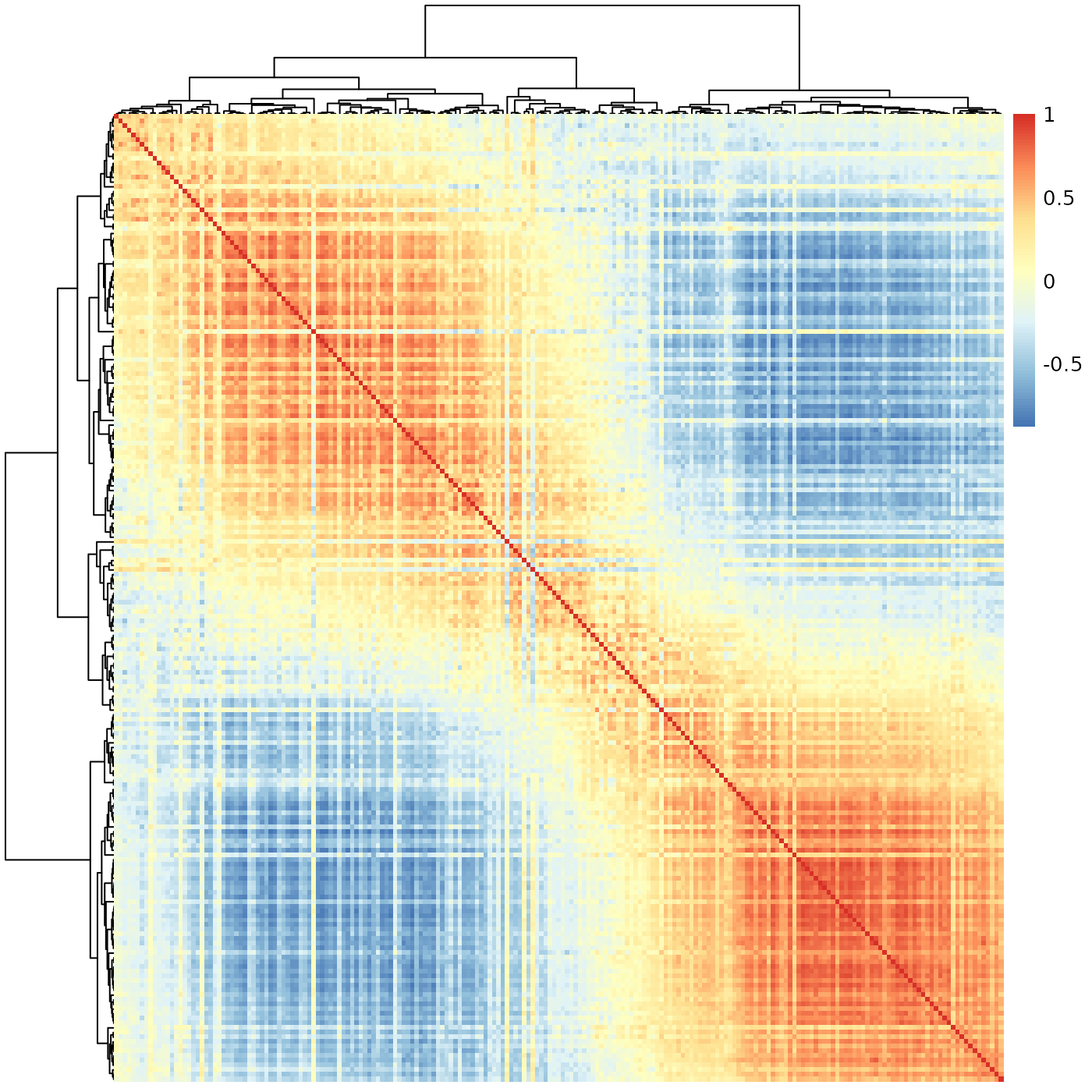This is a simple matrix where each entry is the similarity (correlation) between a pair of batches. Negative correlations were changed to zero to simplify the analysis.

data(meristems)

## Format

A simple square matrix.

## Examples

data(meristems)
similarity <- meristems
similarity[similarity < 0] = 0
slanter::sheatmap(meristems, order_data=similarity, show_rownames=FALSE, show_colnames=FALSE)